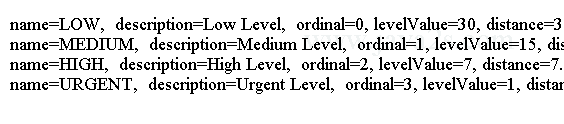# Java Object Oriented Design - Java Data Type

## Adding bodies to an Enum Constant

We can add a different body to each enum constant. The body can have fields and methods.

The body for an enum constant is placed inside braces following its name.

If the enum constant accepts arguments, its body follows its argument list. The syntax for associating a body to an enum constant is as follows:

```<access-modifier> enum <enum-type-name>  {
ENUM_VALUE1  {
// Body for ENUM_VALUE1  goes  here
},
ENUM_VALUE2  {
// Body for ENUM_VALUE2  goes  here
},
ENUM_VALUE3(arguments-list)  {
// Body of  ENUM_VALUE3  goes  here
};

// Other  code  goes  here
}
```

## Example

The following code creates Level enum type with body.

```enum Level {/*ww w .  ja v  a  2  s.c  om*/
LOW("Low Level", 30) {
public double getDistance() {
return 30.0;
}
},
MEDIUM("Medium Level", 15) {
public double getDistance() {
return 15.0;
}
},
HIGH("High Level", 7) {
public double getDistance() {
return 7.0;
}
},
URGENT("Urgent Level", 1) {
public double getDistance() {
return 1.0;
}
};

private int levelValue;
private String description;

private Level(String description, int levelValue) {
this.description = description;
this.levelValue = levelValue;
}

public int getLevelValue() {
return levelValue;
}

@Override
public String toString() {
return this.description;
}

public abstract double getDistance();
}

public class Main {
public static void main(String[] args) {
for (Level s : Level.values()) {
String name = s.name();
String desc = s.toString();
int ordinal = s.ordinal();
int levelValue = s.getLevelValue();
double distance = s.getDistance();
System.out.println("name=" + name + ",  description=" + desc
+ ",  ordinal=" + ordinal + ", levelValue=" + levelValue
+ ", distance=" + distance);
}
}
}
```

Level enum has an abstract method getDistance().

Each instance constant has a body that provides implementation for the getDistance() method.

It has overridden the toString() method in the Enum class.

The code above generates the following result.